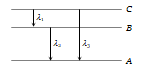The Rutherford $\mathrm{\alpha }$-particle experiment shows that most of the $\mathrm{\alpha }$-particles pass through almost unscattered while some are scattered through large angles. What information does it give about the structure of the atom

(a) Atom is hollow

(b) The whole mass of the atom is concentrated in a small centre called nucleus

(c) Nucleus is positively charged

(d) All the above

Concept Questions :-

Various atomic model

Difficulty Level:

Which of the following is true

(a) Lyman series is a continuous spectrum

(b) Paschen series is a line spectrum in the infrared

(c) Balmer series is a line spectrum in the ultraviolet

(d) The spectral series formula can be derived from the Rutherford model of the hydrogen atom

Concept Questions :-

Spectral series
High Yielding Test Series + Question Bank - NEET 2020

Difficulty Level:

The energy required to knock out the electron in the third orbit of a hydrogen atom is equal to
(a) 13.6 eV           (b) $+\frac{13.6}{9}\mathrm{eV}$
(c) $-\frac{13.6}{3}\mathrm{eV}$       (d) $-\frac{3}{13.6}\mathrm{eV}$

Concept Questions :-

Bohr's model of atom
High Yielding Test Series + Question Bank - NEET 2020

Difficulty Level:

An electron has a mass of . It revolves round the nucleus in a circular orbit of radius $0.529×{10}^{-10}$ metre at a speed of . The magnitude of its linear momentum in this motion is

(a) kg-m/s             (b) $2.0×{10}^{-24}$ kg-m/s
(c) $4.0×{10}^{-24}$ kg-m/s           (d) $4.0×{10}^{-31}$ kg-m/s

Concept Questions :-

Various atomic model
High Yielding Test Series + Question Bank - NEET 2020

Difficulty Level:

The ionization potential for second He electron is
(a) 13.6 eV                 (b) 27.2 eV
(c) 54.4 eV                 (d) 100 eV

Concept Questions :-

Bohr's model of atom
High Yielding Test Series + Question Bank - NEET 2020

Difficulty Level:

The energy required to remove an electron in a hydrogen atom from n = 10 state is
(a) 13.6 eV                   (b) 1.36 eV
(c) 0.136 eV                  (d) 0.0136 eV

Concept Questions :-

Bohr's model of atom
High Yielding Test Series + Question Bank - NEET 2020

Difficulty Level:

Every series of hydrogen spectrum has an upper and lower limit in wavelength. The spectral series which has an upper limit of wavelength equal to 18752 Å is
(a) Balmer series                (b) Lyman series
(c) Paschen series               (d) Pfund series

(Rydberg constant R = $1.097×{10}^{7}$ per metre)

Concept Questions :-

Spectral series
High Yielding Test Series + Question Bank - NEET 2020

Difficulty Level:

The kinetic energy of the electron in an orbit of radius r in hydrogen atom is (e = electronic charge)
(a) $\frac{{\mathrm{e}}^{2}}{{\mathrm{r}}^{2}}$                (b) $\frac{{\mathrm{e}}^{2}}{2\mathrm{r}}$
(c) $\frac{{\mathrm{e}}^{2}}{\mathrm{r}}$                (d) $\frac{{\mathrm{e}}^{2}}{2{\mathrm{r}}^{2}}$

Concept Questions :-

Bohr's model of atom
High Yielding Test Series + Question Bank - NEET 2020

Difficulty Level:

Energy levels A, B, C of a certain atom corresponding to increasing values of energy i.e. ${\mathrm{E}}_{\mathrm{A}}<{\mathrm{E}}_{\mathrm{B}}<{\mathrm{E}}_{\mathrm{C}}$. If  are the wavelengths of radiations corresponding to the transitions C to B, B to A and C to A respectively, which of the following statements is correct(a) ${\mathrm{\lambda }}_{3}={\mathrm{\lambda }}_{1}+{\mathrm{\lambda }}_{2}$                      (b) ${\mathrm{\lambda }}_{3}=\frac{{\mathrm{\lambda }}_{1}{\mathrm{\lambda }}_{2}}{{\mathrm{\lambda }}_{1}+{\mathrm{\lambda }}_{2}}$
(c) ${\mathrm{\lambda }}_{1}+{\mathrm{\lambda }}_{2}+{\mathrm{\lambda }}_{3}=0$                 (d) ${\mathrm{\lambda }}_{3}^{2}={\mathrm{\lambda }}_{1}^{2}+{\mathrm{\lambda }}_{2}^{2}$

Concept Questions :-

Spectral series
High Yielding Test Series + Question Bank - NEET 2020

Difficulty Level:

The angular momentum of electron in nth orbit is given by

(a) nh                 (b) $\frac{\mathrm{h}}{2\mathrm{\pi n}}$
(c) $\mathrm{n}\frac{\mathrm{h}}{2\mathrm{\pi }}$             (d) ${\mathrm{n}}^{2}\frac{\mathrm{h}}{2\mathrm{\pi }}$

Concept Questions :-

Bohr's model of atom